# Problem on Diffraction Grating and Laser Crystallography

Homework Statement:
Please see pictures and text below
Relevant Equations:
This is mostly a conceptual question, but intensity distribution equation for diffraction grating may be helpful.
First question: When shining a laser (##\lambda = 630nm##) through a 2D crystal, diffraction patterns are observed. Why?

Possible reasons:
1. Atoms and the space between them act as diffraction gratings.
2. Bragg Diffraction, although in this case the pattern would be observed on the same side as the crystal?
3. X-Ray diffraction. In XRD, are the emitted photons of the excited electrons emitted in random directions? If so, how can a diffraction pattern be seen?

From this part onwards, I have assumed reason 1 to be the reason why such patterns are observed.

Second question: Why are the following diffraction patterns caused by the following crystals? The pictures shown are square unit cells, which make up the respective crystals. Each unit cell of the crystal contains atom(s), which is/are the black square.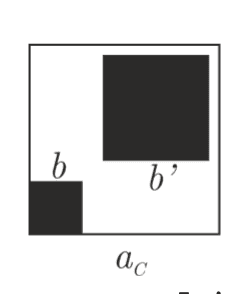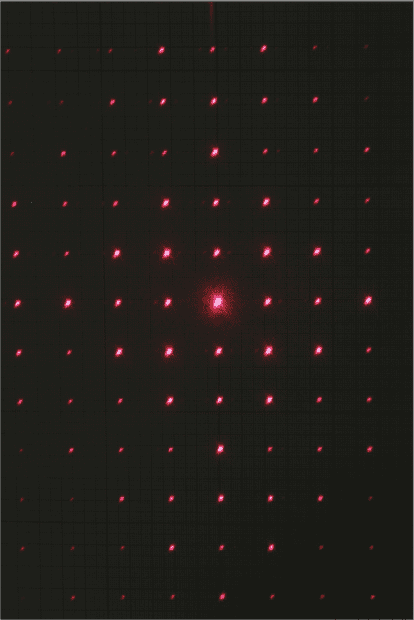- The big atom is displaced vertically from the small atom, so shouldn't the diffraction pattern caused by the big atom be displaced upwards?
Possible reason: The big atom allows so little light to pass through that even the maxima is not visible, due to extremely low intensities, so the entire pattern is caused by the small atom.
- Why is there this "kite" shaped pattern? Without the big atom, the diffraction pattern is a "square" shaped pattern. (see Figure 1 below)
- Would the diagonal of the above crystal form a diffraction grating, although with differing slit width? If so, why is the diffraction pattern of such a grating not visible, or does it overlap with the vertical and horizontal diffraction pattern?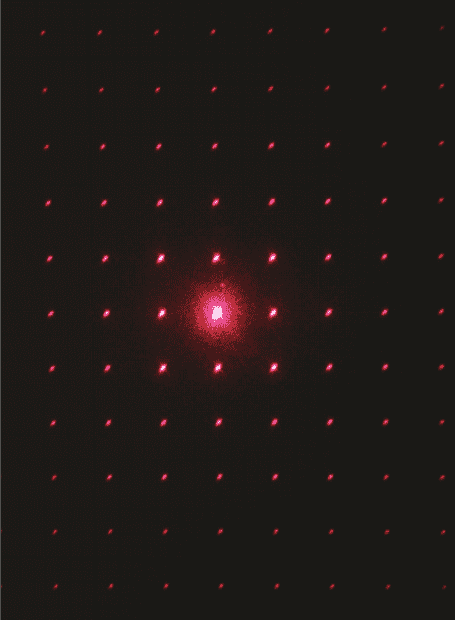<-- Figure 1

I also have some questions about why the crystal on the right results in the left pattern.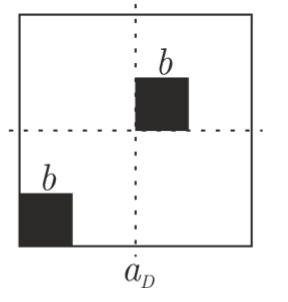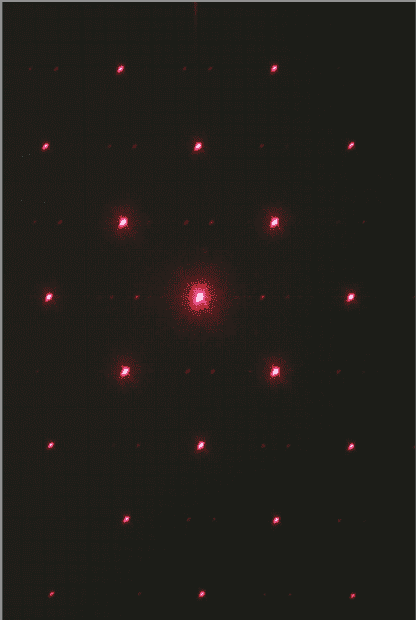- In this case, 1 of the atoms is displaced upwards and rightwards. To me, it is expected that there is a corresponding displacement in the diffraction pattern. (Contrast with above case)

- If you look very carefully, there are pairs of dots everywhere in the above pattern. What causes them?
Possible reason:
The dots are the secondary maxima of the diffraction grating formed by the horizontal row of atoms. However, why the vertical diffraction grating does not cause these pairs of dots is unclear.

Thank you for reading through such a long post. If anything is unclear, you can refer to: https://onedrive.live.com/?authkey=...!147488&parId=85D7064386310CC9!147487&o=OneUp
for the original problem.

haruspex
Homework Helper
Gold Member
2020 Award
My interpretation of the atom diagrams is that you should think of the whole square being repeated indefinitely in both directions. With that view, there is no "upward displacement".
In "figure 1" (second image), the atomic arrangement is a simple rectangular grid:
o o o
o o o
o o o
In the third instance, it looks like this:
o—-o—-o
—o—-o—-o
o—-o—-o
I.e. the same but rotated 45 degrees.
(Hyphens inserted to stop the editor throwing away some spaces.)
What do you see if you write out the pattern for the two unequal atoms?

Last edited:
•phantomvommand
hutchphd
Homework Helper
Homework Statement:: Please see pictures and text below
Relevant Equations:: This is mostly a conceptual question, but intensity distribution equation for diffraction grating may be helpful.

When shining a laser (λ=630nm) through a 2D crystal, diffraction patterns are observed. Why?
First things first.
You cannot see diffraction from atomic crystals using a red laser. The spacing of the lattice must be similar in size to the wavelength. Light wavelengths are thousands of Angstroms atomic spacings are Angstroms.
You therefore need x-rays to look at atomic crystals using various techniques. Bragg and Laue diffraction are the most common. Each spot seen corresponds to coherent reflection (or re-emission if you like) from many atoms.
Try some Wkipedia ?

•phantomvommand
My interpretation of the atom diagrams is that you should think of the whole square being repeated indefinitely in both directions. With that view, there is no "upward displacement".
In "figure 1" (second image), the atomic arrangement is a simple rectangular grid:
o o o
o o o
o o o
In the third instance, it looks like this:
o—-o—-o
—o—-o—-o
o—-o—-o
I.e. the same but rotated 45 degrees.
(Hyphens inserted to stop the editor throwing away some spaces.)
What do you see if you write out the pattern for the two unequal atoms?
Thanks for this. The “upward displacement” I’m referring to, is that of the diffraction pattern observed, not of the atoms/unit cells. I am also assuming the unit cells are extended along the vertical and horizontal directions (and they should be).
The thing is, if you look at the 2nd unit cell, which has 1 large atom in it and 1 small atom, you would expect that in the entire Crystal (ie unit cell extended in xy axes) would have a column and row of large atoms displaced upwards and rightwards respectively from the small atoms. This results in the diffraction pattern being displaced accordingly. Yet, if you look at the diffraction pattern, there is no such displacement observed.

Further elaboration:
The following is the structure of the crystal, O represents large atoms, x represents small atoms.
4 —-O——-O—
3 x——x——-x—-
2 —-O——O——O
1 x——x——-x—-
1 2 3 4 5 6

The box bounded by 1122 corresponds to 1 unit cell, with 1 large and 1 small atom.
The diffraction due to the the “grating” formed by the “x” atoms in column 1, should be displaced from the diffraction formed by the “grating” made up of “O” atoms in column 2. A similar argument applies row-wise/horizontally.

The above theory how I reasoned the diffraction pattern of the last unit cell structure shown, would have vertical and horizontal maxima displaced from each other. The following diagram represents the diffraction pattern of the last crystal:
4 —x—x—x
3 x—-x—x-
2 —x—x—x
1 x—-x—x-
1 2 3 4 5 6

Column 1 is due to the 1st column of atoms, column 2 is due to the 2nd column of displaced atoms; likewise for rows.

First things first.
You cannot see diffraction from atomic crystals using a red laser. The spacing of the lattice must be similar in size to the wavelength. Light wavelengths are thousands of Angstroms atomic spacings are Angstroms.
You therefore need x-rays to look at atomic crystals using various techniques. Bragg and Laue diffraction are the most common. Each spot seen corresponds to coherent reflection (or re-emission if you like) from many atoms.
Try some Wkipedia ?
Thanks for this. Somehow, I tried it out in real life with a red laser, and the patterns are really observed. However, I suspect they used a diffraction grating instead, particularly since they already instructed us to assume the effect of the atoms is that of setting up a “grating”, where the space between atoms act as “slits”.

Assuming it were a diffraction grating (most probably a 2D grating), do you have any idea how the following patterns could be observed?

haruspex
Homework Helper
Gold Member
2020 Award
The diffraction due to the the “grating” formed by the “x” atoms in column 1, should be displaced from the diffraction formed by the “grating” made up of “O” atoms in column 2.
You seem to be thinking of the image as though it is a blown up photo of the lattice.
The displacement of the image would only be the same as the displacement of one set of atoms in relation to the other, i.e. the interatomic distance. You are not going to notice that! Forget displacements.

You should review the principles of diffraction and how these lead to the placement and intensities of the spots. But if you accept that the simple rectangular grid case leads to "figure 1" it isn't hard to see how the other two may happen.
In the third example, the atomic grid is the same as in "figure 1" but rotated 45 degrees. Consequently the image is rotated 45 degrees.

The first example is a bit of a hybrid. On the one hand, the complete set of atoms is like the third example, leading to a diamond pattern of intensities. On the other, just looking at one of the two families of atoms it looks like the second example, "figure 1". So if there is a reason why the larger atoms, say, will have a dominant effect then you would expect the image to look like a hybrid of the two cases.
Now, that is just a hand-waving explanation of how you could get what you see; it is in no way proof.

•phantomvommand
You seem to be thinking of the image as though it is a blown up photo of the lattice.
The displacement of the image would only be the same as the displacement of one set of atoms in relation to the other, i.e. the interatomic distance. You are not going to notice that! Forget displacements.

You should review the principles of diffraction and how these lead to the placement and intensities of the spots. But if you accept that the simple rectangular grid case leads to "figure 1" it isn't hard to see how the other two may happen.
In the third example, the atomic grid is the same as in "figure 1" but rotated 45 degrees. Consequently the image is rotated 45 degrees.

The first example is a bit of a hybrid. On the one hand, the complete set of atoms is like the third example, leading to a diamond pattern of intensities. On the other, just looking at one of the two families of atoms it looks like the second example, "figure 1". So if there is a reason why the larger atoms, say, will have a dominant effect then you would expect the image to look like a hybrid of the two cases.
Now, that is just a hand-waving explanation of how you could get what you see; it is in no way proof.
Thanks a lot for this. If the vertical displacement between vertical columns of atoms (ie vertical diffraction gratings) is so small as to be indiscernible, why would 2 vertical diffraction grating patterns be spaced a few centimetres apart, since the horizontal distance between 2 vertical columns of atoms is also too small?

haruspex
Homework Helper
Gold Member
2020 Award
If the vertical displacement between vertical columns of atoms (ie vertical diffraction gratings) is so small as to be indiscernible, why would 2 vertical diffraction grating patterns be spaced a few centimetres apart, since the horizontal distance between 2 vertical columns of atoms is also too small?
Because the diffraction pattern is not simply a photo of the grid.
The separation of the dots in the image is related to the separation of the atoms via Bragg's Law, ##2d\sin(\theta_n)=n\lambda##, where d is the atomic separation. The position of lines in the image is given by ##y_n=D\tan(\theta_n)##, where D is the distance to the screen.
Thus, decreasing d increases the separation.
If we displace the entire crystal grid by d, the image is displaced by d, which is utterly insignificant compared with ##\frac Dd\lambda##.
If we displace the grid of large atoms relative to the grid of small atoms then it will change the image because of the change in interatomic distances, but the change will not consist of a simple displacement.

•phantomvommand
Because the diffraction pattern is not simply a photo of the grid.
The separation of the dots in the image is related to the separation of the atoms via Bragg's Law, ##2d\sin(\theta_n)=n\lambda##, where d is the atomic separation. The position of lines in the image is given by ##y_n=D\tan(\theta_n)##, where D is the distance to the screen.
Thus, decreasing d increases the separation.
If we displace the entire crystal grid by d, the image is displaced by d, which is utterly insignificant compared with ##\frac Dd\lambda##.
If we displace the grid of large atoms relative to the grid of small atoms then it will change the image because of the change in interatomic distances, but the change will not consist of a simple displacement.
I highly doubt this is Bragg Diffraction. The measured values of the atom and period size are of the order 10^-6, which is much larger than typical atom distances. Furthermore, the light was shone through a very thin, shaded area printed on a flimsy piece of thin plastic, so I think that the atoms in the “2D crystal” are actually completely covered parts between slits in a 2D diffraction grating. Apologies for not mentioning this earlier.

I am quite confused, because the setup is exactly like that of a normal diffraction:

laser —> sample ——————> screen

If it were Bragg diffraction, such a setup would not yield results, since reflection is involved?

The effect of such a “2D grating” is also not clear. I have been imagining it’s Resulting pattern as an addition of patterns due to diffraction gratings vertically and horizontally, but I think this is incorrect. I think it would be helpful if you could elaborate more on how patterns are even formed, such as where the light is diffracted etc. (I did not find good explanations online :/ )

I understand your previous explanation of seeing the grating’s pattern as a whole, resulting in the rotation for the last sample. However, isn’t the sample with 1 big and 1 small atom also rotated? Why is only the diamond shaped intensity character kept?

thanks for all your help so far!

hutchphd
Homework Helper
My guess is that this is a hologram (or holographic grating....) and corresponds io a 2D transmission grating. It is the same physics.

•phantomvommand
haruspex
Homework Helper
Gold Member
2020 Award
I highly doubt this is Bragg Diffraction. The measured values of the atom and period size are of the order 10^-6, which is much larger than typical atom distances. Furthermore, the light was shone through a very thin, shaded area printed on a flimsy piece of thin plastic, so I think that the atoms in the “2D crystal” are actually completely covered parts between slits in a 2D diffraction grating. Apologies for not mentioning this earlier.

I am quite confused, because the setup is exactly like that of a normal diffraction:

laser —> sample ——————> screen

If it were Bragg diffraction, such a setup would not yield results, since reflection is involved?

The effect of such a “2D grating” is also not clear. I have been imagining it’s Resulting pattern as an addition of patterns due to diffraction gratings vertically and horizontally, but I think this is incorrect. I think it would be helpful if you could elaborate more on how patterns are even formed, such as where the light is diffracted etc. (I did not find good explanations online :/ )

I understand your previous explanation of seeing the grating’s pattern as a whole, resulting in the rotation for the last sample. However, isn’t the sample with 1 big and 1 small atom also rotated? Why is only the diamond shaped intensity character kept?

thanks for all your help so far!
As @hutchphd mentions, the exact type of diffraction is largely irrelevant.
Because of the reflection involved in Bragg diffraction, there is the factor 2 in ##2d\sin(\theta_n)=n\lambda##, where d is the interplanar depth.
For a single slit width d, ##d\sin(\theta_n)=n\lambda##.

What you appear to have is a 2D grating. It seems reasonable that this would produce a rectangular pattern of dots in the simplest case, but even here I am unsure. That would follow from considering nearest neighbours, but what about the diagonal direction? If nearest neighbours produce a grid with angular separation ##\frac{\lambda}d##, wouldn't second-nearest (i.e. diagonally adjacent) neighbours produce a (fainter?) grid with angular separation ##\frac{\lambda}{d\sqrt 2}## in the diagonal direction?

For the purpose of your question, we also need to consider the intensities. This is even more complicated - see e.g. http://hyperphysics.phy-astr.gsu.edu/hbase/phyopt/sinint.html.

In short, I am not able to help any further. My handwaving explanation in post #6 is my best shot.

•phantomvommand